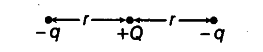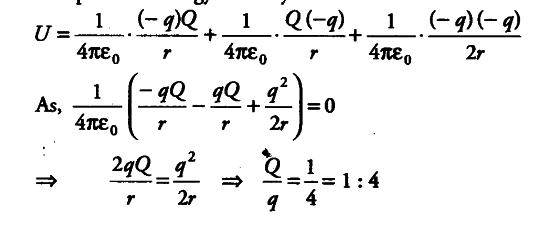# Three charges - q, + Q and - q are placed at equal distance oh straight line

Three charges - q, + Q and - q are placed at equal distance oh straight line. If the potential energy of the system of the three charges is zero, then what is the ratio of Q: q ?

Let the three charges be located as shown in the figure.The potential energy of the system be